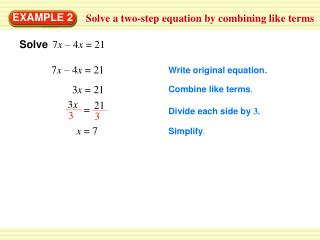Download PresentationSolve a two-step equation by combining like terms

Solve a two-step equation by combining like terms

Download PresentationSolve a two-step equation by combining like terms

- - - - - - - - - - - - - - - - - - - - - - - - - - - E N D - - - - - - - - - - - - - - - - - - - - - - - - - - -
Presentation Transcript

1. = 3x 21 3 3 EXAMPLE 2 Solve a two-step equation by combining like terms Solve7x – 4x = 21 7x – 4x = 21 Write original equation. 3x = 21 Combine like terms. Divide each side by 3. x = 7 Simplify.

2. SOLUTION STEP1 Write an equation for the function. Let xbe the input and ybe the output STEP 2 Solve the equation for xwhen y =17. EXAMPLE 3 Find an input of a function The output of a function is 3 less than 5 times the input. Find the input when the output is 17. y = 5x – 3 yis 3 less than 5 times x.

3. 20 5x = 5 5 ANSWER An input of4 produces an output of17. EXAMPLE 3 Find an input of a function y = 5x – 3 Write original function. 17 = 5x – 3 Substitute17 fory. 17 + 3= 5x – 3 +3 Add 3 to each side. 20 = 5x Simplify. Divide each side by5. 4 = x Simplify.

4. ? 17 = 5(4) – 3 ? 17 = 20– 3 17 = 17 EXAMPLE 3 Find an input of a function CHECK y = 5x– 3 Write original function. Substitute 17 for yand 4 for x. Multiply 5 and 4. Simplify. Solution checks.

5. ANSWER 4 for Examples 2 and 3 GUIDED PRACTICE Solve the equation. Check your solution. 4. 4w + 2w = 24

6. ANSWER 7 for Examples 2 and 3 GUIDED PRACTICE Solve the equation. Check your solution. 5. 8t – 3t = 35

7. ANSWER 4 for Examples 2 and 3 GUIDED PRACTICE Solve the equation. Check your solution. 6. –16= 5d – 9d

8. 7. The output of a function is 5 more than –2 times the input. Find the input when the output is 11. ANSWER –3 for Examples 2 and 3 GUIDED PRACTICE

9. 8. The output of a function is 4 less than 4 times the input. Find the input when the output is 3 3 ANSWER 1 4 for Examples 2 And 3 GUIDED PRACTICE